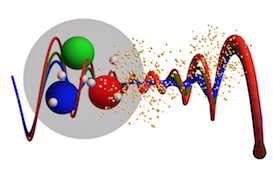#13th International workshop on Multiple Partonic Interactions at the LHC

14-18 November 2022

## Back-to-back di-$\pi^{0}$ azimuthal correlations at forward rapidities at STAR

15 Nov 2022, 15:40
20m
WG4: Small-x and Diffraction

### Speaker

Xiaoxuan Chu (BNL)

### Description

The back-to-back di-$\pi^{0}$ correlation functions in forward directions have been observed to be suppressed in $p(d)+$A collisions relative to $p$$+$$p$ collisions. The nonlinear gluon dynamics in nuclei is one of the possible mechanisms responsible for the suppression. In addition, theorists pointed out that the double-parton scattering (DPS) can be an alternative explanation of the suppression in $d+$A collisions. During the 2015 and 2016 RHIC runs, STAR collected data with the Forward Meson Spectrometer (FMS, 2.6 < $\eta$ < 4.0) in $p$$+$$p$, $p+$Al, $p$+Au, and $d$+Au collisions at $\sqrt{s_{\rm{_{NN}}}}=200$ GeV. The recorded datasets enable the measurements of di-$\pi^{0}$ azimuthal correlations in $p$$+$$p$ and $p+$A collisions with different mass numbers. Meanwhile, a comparison of the correlations in $d+$Au and $p+$Au collisions provides an opportunity to study the impact of DPS. In this talk, we will present the results on di-$\pi^{0}$ correlations at forward rapidities in $p$$+$$p$ and $p+$A collisions, together with the preliminary results in $d$+Au collisions.

### Primary author

Xiaoxuan Chu (BNL)

### Presentation Materials

###### Your browser is out of date!

Update your browser to view this website correctly. Update my browser now

×